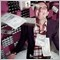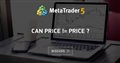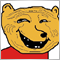# New MetaTrader 4 build 1160 - page 424

I think these codes may be a clue which the "union" will generate stack overflow:

```struct SDecimal
{
double _db0;
double _db1;
};

//---
union decimal
{
SDecimal _dec;

decimal operator=( const double _double );
decimal operator=( const decimal &_decimal );
decimal operator=( const SDecimal &_sdec );
};

//---
decimal decimal::operator=( const double _double )
{
_dec._db0 = _double;

return this;
}

//---
decimal decimal::operator=( const decimal &_decimal )
{
_dec = _decimal._dec;

return this;
}

decimal decimal::operator=( const SDecimal &_sdec )
{
return this;
}

void OnTick(void)
{
decimal a, b, c, d;

a = b = c = d = 0.0;
}```40775

googollien:

I think these codes may be a clue which the "union" will generate stack overflow:

MT4 (backtest) report an error and doesn't crash. It does the same under build 1090.

2018.12.16 12:56:06.961    2016.04.13 22:00:00  Testing pass stopped due to a critical error in the EA
2018.12.16 12:56:06.961    2016.04.13 22:00:00  Stack overflow in 'D:\MT4\Alpari\MQL4\Experts\Forum\294630.ex4'24

Alain Verleyen:

MT4 (backtest) report an error and doesn't crash. It does the same under build 1090.

2018.12.16 12:56:06.961    2016.04.13 22:00:00  Testing pass stopped due to a critical error in the EA
2018.12.16 12:56:06.961    2016.04.13 22:00:00  Stack overflow in 'D:\MT4\Alpari\MQL4\Experts\Forum\294630.ex4'

Thanks for your reply, the similar thing, compiler complain some weird error:

```struct SDecimal
{
double _db0;
double _db1;
};

//---
union decimal
{
SDecimal _dec;

decimal operator=( const double _double );
decimal operator=( const decimal &_decimal );
decimal operator=( const SDecimal &_sdec );
};

//---
decimal decimal::operator=( const double _double )
{
_dec._db0 = _double;

return this;
}

//---
decimal decimal::operator=( const decimal &_decimal )
{
_dec = _decimal._dec;

return this;
}

decimal decimal::operator=( const SDecimal &_sdec )
{
return this;
}

void OnTick(void)
{
decimal a, b, c, d;

d._dec._db0 = 0.0;
a._dec = b._dec = c._dec = d._dec;
}```

'operator=' - expression of 'void' type is illegal MACD Sample.mq4 66 26

'operator=' - object required MACD Sample.mq4 66 17

It works for me and I still have some important codes to write.

I really need it caused my project is really reaching the deadline.24

Alain Verleyen:
If you want it to be fixed, you probably have to provide a testcase code to reproduce the problem. (maybe just remove the trading logic or something).

I am pretty sure the MT4 crashed in strategy tester for 3 months data issue must be related with "operator overload" of "union".

These are CRASHED codes:

```//################### CRASHED CODES #############
struct SDecimal
{
double _db0;
double _db1;
};

//---
union decimal
{
SDecimal _dec;

decimal operator=( const double _double );

decimal operator+=( const double dbl );
decimal operator+( const double dbl ) const;
decimal operator-=( const double dbl );
decimal operator-( const double dbl ) const;
decimal operator/( const double dbl ) const;
decimal operator/( const double _int ) const;
};
```

BUT, when I instead those operators with NORMAL functions and using the SAME BODY CODES, then MT4 gets stable and no crash any more:

```//\$\$\$\$\$\$\$\$\$\$\$\$\$\$\$\$\$\$\$\$ WORKS CODES \$\$\$\$\$\$\$\$\$\$\$\$
struct SDecimal
{
double _db0;
double _db1;
};

//---
union decimal
{
SDecimal _dec;

decimal Double( const double _double );         //decimal operator=( const double _double );

decimal Add( const double dbl );                //decimal operator+( const double dbl ) const;
decimal Sub( const double dbl );                //decimal operator-( const double dbl ) const;
decimal AddEqu( const double dbl );             //decimal operator+=( const double dbl );
decimal SubEqu( const double dbl );             //decimal operator-=( const double dbl );

double Div( const int _int );                   //decimal operator/( const double _int ) const;
double Div( const double dbl );                 //decimal operator/( const double dbl ) const;
};```

Seems like those "operator" functions have some memory leak or something else.40775

googollien:

I am pretty sure the MT4 crashed in strategy tester for 3 months data issue must be related with "operator overload" of "union".

These are CRASHED codes:

BUT, when I instead those operators with NORMAL functions and using the SAME BODY CODES, then MT4 gets stable and no crash any more:

Seems like those "operator" functions have some memory leak or something else.

If you don't provide code that I can compile and test, I can't help.

If you don't want auto-update you have to block the updates yourself.24

Alain Verleyen:

If you don't provide code that I can compile and test, I can't help.

If you don't want auto-update you have to block the updates yourself.

If you insisted, these codes may not reproduce the issue, because the times to do calculations and calls may not be so few:

```//---
union ULongLong
{
bool _bool[ 16 ];
uchar _uchar[ 16 ];
ushort _ushort[ 8 ];
short _short[ 8 ];
uint _uint[ 4 ];
int _int[ 4 ];
ulong _ulong[ 2 ];
long _long[ 2 ];
double _double[ 2 ];
};

struct SDecimal
{
double _db0;
double _db1;
};

//---
union decimal
{
ULongLong _ulonglong;
SDecimal _dec;

decimal operator=( const double _double );

decimal operator+=( const double dbl );
decimal operator+( const double dbl ) const;
decimal operator-=( const double dbl );
decimal operator-( const double dbl ) const;
double operator/( const double dbl );

double ToDouble() const;

decimal( int value );
decimal( double value );
decimal() {};
};

//---
decimal decimal::operator=( const double _double )
{
_dec._db0 = _double;

return this;
}

//---
decimal decimal::operator+=( const double dbl )
{
decimal val( dbl );

return this;
}

//---
decimal decimal::operator+( const double dbl ) const
{
decimal ans, val( dbl );

return ans;
}

//---
decimal decimal::operator-=( const double dbl )
{
decimal val( dbl );

return this;
}

//---
decimal decimal::operator-( const double dbl ) const
{
decimal ans, val( dbl );

return ans;
}

//---
double decimal::operator/( const double dbl )
{
decimal ans, val( dbl );

return ans.ToDouble();
}

//---
decimal::decimal( int value )
{
_ulonglong._int[ 0 ] = value;
}

//---
decimal::decimal( double value )
{
_dec._db0 = value;
}

//---
double decimal::ToDouble() const
{
return _dec._db0;
}

decimal a, b, c, d;

void OnTick(void)
{
for( int i = 0; i < 500; i++ )
{
a = 10.0;
b += 1.7;
c = a + 2.5;
d = c - 0.7;
d -= 0.3;
d = d / 1.0;
}
}```

Such situation must be caused by the MQL compiler or inner executing functions where to do "operator" of the "union",
but.... maybe just fine to keep this bug, I had found other way to do it, and it works. i.e. I will avoid to do any "operator"
codes in the future.

This part seems never be done and always having something wrong with syntax or compiler since the "union" was supported.

Thanks anyway.276

googollien:

If you want to block automatic updates, just find folder "webinstall" and change security, adding "deny" permission to user "everyone".

This will block the folder to everyone, including MT4, which will be unable to auto-update.

Folder is located in roaming user profile AppData\Roaming\MetaQuotes\WebInstall

I still have build 1090 archive just in case.31

Bug report.

There is a way to compare doubles that for a long time has been recommended by senior forum members.

See https://www.mql5.com/en/forum/136997/page7

This piece of code contains "return (-1);", and with this version it does no longer go through the compiler since the function is supposed to return a boolean.

I have this function in hundreds of EAs and indicators, spread to hundreds of clients. And I am sure I am not the only one since it has repeatedly been recommended on the forums. With this version, each and everyone of those EAs and indicators will no longer compile. Please fix change this back in a new build, otherwise there will be thousands and thousands of EAs out there that do no longer work.

```#define LT    0
#define GT    1
#define GTE   2
#define LTE   3
#define EQ    4
#define NEQ   5

bool doublecomp(double a,int type,double b){
// See https://forum.mql4.com/45053/page4
// 0 compare doesn't need this function
switch(type){
case LT: return(b-a>Point/2.);
case GT: return(a-b>Point/2.);
case EQ: return(!(MathAbs(a-b)>Point/2.));
case NEQ: return(MathAbs(a-b)>Point/2.);
default: return(-1); // Added - needed to avoid compile error about not all control paths returning a value
}
}```Can price != price ?
• 2014.06.19
• www.mql5.com
I'm trying to understand something strange that I am seeing so I can better code round it in the future...24

Philippe Pauleau:

If you want to block automatic updates, just find folder "webinstall" and change security, adding "deny" permission to user "everyone".

This will block the folder to everyone, including MT4, which will be unable to auto-update.

Folder is located in roaming user profile AppData\Roaming\MetaQuotes\WebInstall

I still have build 1090 archive just in case.

Hello, Philippe, thanks for kindly sharing information. :)

I am cool with the 1160 build now, just NEVER USE OPERATOR OVERLOAD / OVERLAP FUNCTIONS, then things go well.1080

Bug report.

There is a way to compare doubles that for a long time has been recommended by senior forum members.

See https://www.mql5.com/en/forum/136997/page7

This piece of code contains "return (-1);", and with this version it does no longer go through the compiler since the function is supposed to return a boolean.

I have this function in hundreds of EAs and indicators, spread to hundreds of clients. And I am sure I am not the only one since it has repeatedly been recommended on the forums. With this version, each and everyone of those EAs and indicators will no longer compile. Please fix change this back in a new build, otherwise there will be thousands and thousands of EAs out there that do no longer work.